# findsignal

Find signal location using similarity search

## Syntax

``````[istart,istop,dist] = findsignal(data,signal)``````
``````[istart,istop,dist] = findsignal(data,signal,Name,Value)``````
``findsignal(___)``

## Description

example

``````[istart,istop,dist] = findsignal(data,signal)``` returns the start and stop indices of a segment of the data array, `data`, that best matches the search array, `signal`. The best-matching segment is such that `dist`, the squared Euclidean distance between the segment and the search array, is smallest. If `data` and `signal` are matrices, then `findsignal` finds the start and end columns of the region of `data` that best matches `signal`. In that case, `data` and `signal` must have the same number of rows.```

example

``````[istart,istop,dist] = findsignal(data,signal,Name,Value)``` specifies additional options using name-value pair arguments. Options include the normalization to apply, the number of segments to report, and the distance metric to use.```

example

````findsignal(___)` without output arguments plots `data` and highlights any identified instances of `signal`. If the arrays are real vectors, the function displays `data` as a function of sample number.If the arrays are complex vectors, the function displays `data` on an Argand diagram.If the arrays are real matrices, the function uses `imagesc` to display `signal` on a subplot and `data` with the highlighted regions on another subplot.If the arrays are complex matrices, the function plots their real and imaginary parts in the top and bottom half of each image. ```

## Examples

collapse all

Generate a data set consisting of a 5 Hz Gaussian pulse with 50% bandwidth, sampled for half a second at a rate of 1 kHz.

```fs = 1e3; t = 0:1/fs:0.5; data = gauspuls(t,5,0.5);```

Create a signal consisting of one-and-a-half cycles of a 10 Hz sinusoid. Plot the data set and the signal.

```ts = 0:1/fs:0.15; signal = cos(2*pi*10*ts); subplot(2,1,1) plot(t,data) title('Data') subplot(2,1,2) plot(ts,signal) title('Signal')```Find the segment of the data that has the smallest squared Euclidean distance to the signal. Plot the data and highlight the segment.

```figure findsignal(data,signal)```Add two clearly outlying sections to the data set. Find the segment that is closest to the signal in the sense of having the smallest absolute distance.

```dt = data; dt(t>0.31&t<0.32) = 2.1; dt(t>0.32&t<0.33) = -2.1; findsignal(dt,signal,'Metric','absolute')```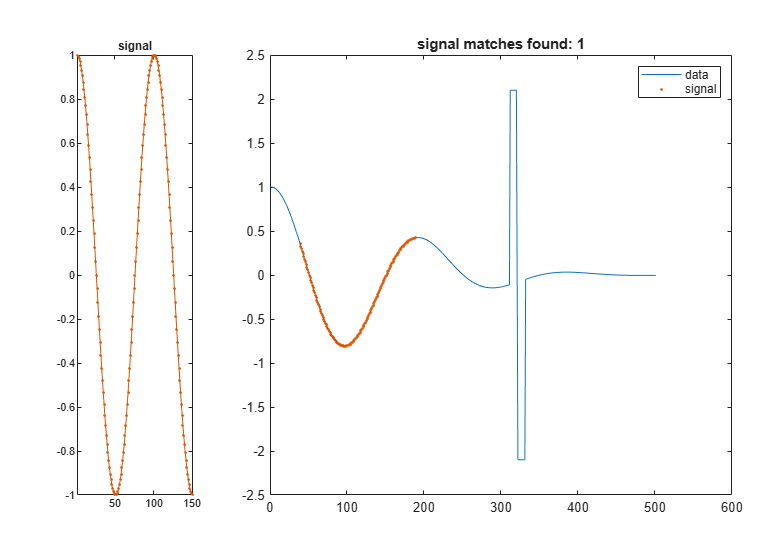Let the x-axes stretch if the stretching results in a smaller absolute distance between the closest data segment and the signal.

`findsignal(dt,signal,'TimeAlignment','dtw','Metric','absolute')`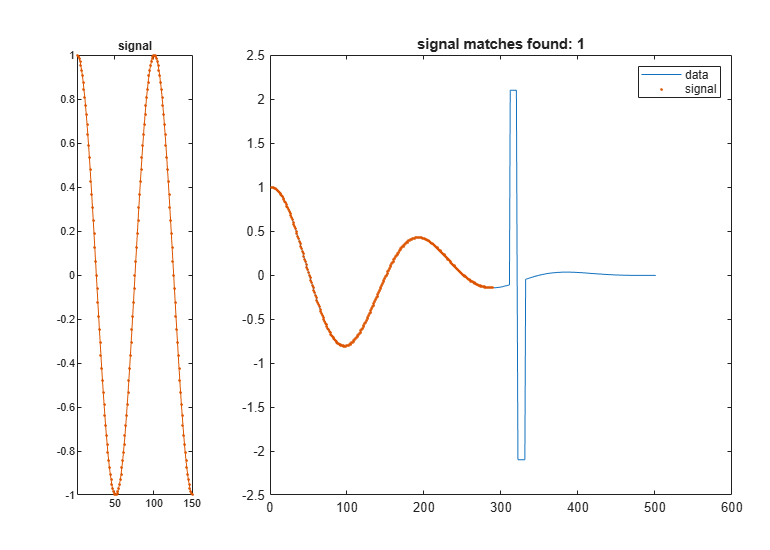Add two more outlying sections to the data set.

```dt(t>0.1&t<0.11) = 2.1; dt(t>0.11&t<0.12) = -2.1; findsignal(dt,signal,'TimeAlignment','dtw','Metric','absolute')```Find the two data segments closest to the signal.

```findsignal(dt,signal,'TimeAlignment','dtw','Metric','absolute', ... 'MaxNumSegments',2)```Go back to finding one segment. Choose `'edr'` as the x-axis stretching criterion. Select an edit distance tolerance of 3. The edit distance between nonmatching samples is independent of the actual separation, making `'edr'` robust to outliers.

```findsignal(dt,signal,'TimeAlignment','edr','EDRTolerance',3, ... 'Metric','absolute')```Repeat the calculation, but now normalize the data and the signal.

• Define a moving window with 10 samples to either side of each data and signal point.

• Subtract the mean of the data in the window and divide by the local standard deviation.

Find the normalized data segment that has the smallest absolute distance to the normalized signal. Display the unnormalized and normalized versions of the data and the signal.

```findsignal(dt,signal,'TimeAlignment','edr','EDRTolerance',3, ... 'Normalization','zscore','NormalizationLength',21, ... 'Metric','absolute','Annotate','all')```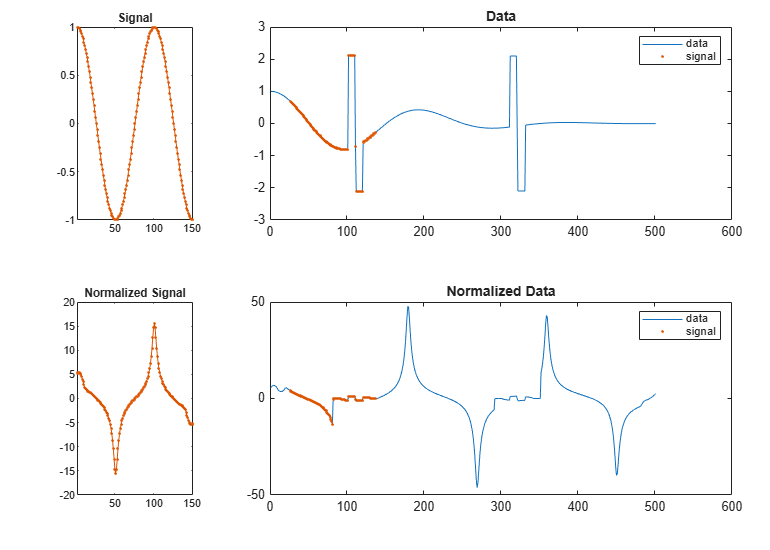Generate a random data array where:

• The mean is constant in each of seven regions and changes abruptly from region to region.

• The standard deviation is constant in each of five regions and changes abruptly from region to region.

```lr = 20; mns = [0 1 4 -5 2 0 1]; nm = length(mns); vrs = [1 4 6 1 3]/2; nv = length(vrs); v = randn(1,lr*nm*nv); f = reshape(repmat(mns,lr*nv,1),1,lr*nm*nv); y = reshape(repmat(vrs,lr*nm,1),1,lr*nm*nv); t = v.*y+f;```

Plot the data, highlighting the steps of its construction. Display the mean and standard deviation of each region.

```subplot(2,2,1) plot(v) title('Original') xlim([0 700]) subplot(2,2,2) plot([f;v+f]') title('Means') xlim([0 700]) text(lr*nv*nm*((0:1/nm:1-1/nm)+1/(2*nm)),-7*ones(1,nm),num2str(mns'), ... 'HorizontalAlignment',"center") subplot(2,2,3) plot([y;v.*y]') title('STD') xlim([0 700]) text(lr*nv*nm*((0:1/nv:1-1/nv)+1/(2*nv)),-7*ones(1,nv),num2str(vrs'), ... 'HorizontalAlignment',"center") subplot(2,2,4) plot(t) title('Final') xlim([0 700])```Create a random signal with a mean of zero and a standard deviation of 1/2. Find and display the segment of the data array that best matches the signal.

```sg = randn(1,2*lr)/2; findsignal(t,sg)```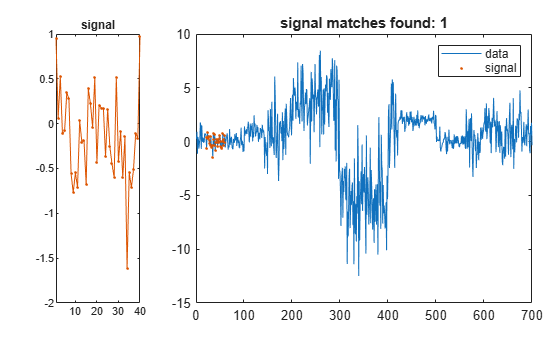Create a random signal with a mean of zero and a standard deviation of 2. Find and display the segment of the data array that best matches the signal.

```sg = randn(1,2*lr)*2; findsignal(t,sg)```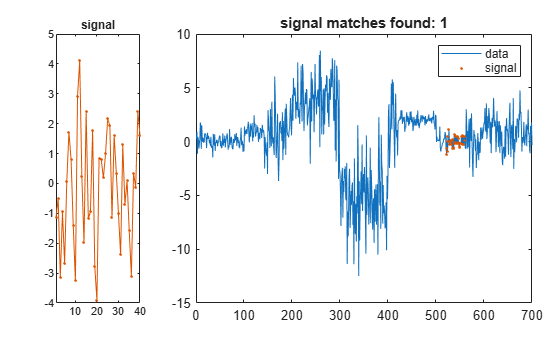Create a random signal with a mean of 2 and a standard deviation of 2. Find and display the segment of the data array that best matches the signal.

```sg = randn(1,2*lr)*2+2; findsignal(t,sg)```Create a random signal with a mean of -4 and a standard deviation of 3. Find and display the segment of the data array that best matches the signal.

```sg = randn(1,2*lr)*3-4; findsignal(t,sg)```Repeat the calculation, but this time subtract the mean from both the signal and the data.

`findsignal(t,sg,'Normalization','zscore','Annotate','all')`Devise a typeface that resembles the output of early computers. Use it to write the word MATLAB®.

```rng default chr = @(x)dec2bin(x')-48; M = chr([34 34 54 42 34 34 34]); A = chr([08 20 34 34 62 34 34]); T = chr([62 08 08 08 08 08 08]); L = chr([32 32 32 32 32 32 62]); B = chr([60 34 34 60 34 34 60]); MATLAB = [M A T L A B];```

Corrupt the word by repeating random columns of the letters and varying the spacing. Show the original word and three corrupted versions.

```c = @(x)x(:,sort([1:6 randi(6,1,2)])); subplot(4,1,1,'XLim',[0 60]) spy(MATLAB) xlabel('') ylabel('Original') for kj = 2:4 subplot(4,1,kj,'XLim',[0 60]) spy([c(M) c(A) c(T) c(L) c(A) c(B)]) xlabel('') ylabel('Corrupted') end```Generate one more corrupted version of the word. Search for a noisy version of the letter "A." Display the distance between the search array and the data segment closest to it. The segment spills into the "T" because the horizontal axes are rigid.

```corr = [c(M) c(A) c(T) c(L) c(A) c(B)]; sgn = c(A); [ist,ind,dst] = findsignal(corr,sgn); clf subplot(2,1,1) spy(sgn) subplot(2,1,2) spy(corr) chk = zeros(size(corr)); chk(:,ist:ind) = corr(:,ist:ind); hold on spy(chk,'*k') hold off````dst`
```dst = 11 ```

Allow the horizontal axes to stretch. The closest segment is the intersection of the search array and the first instance of "A." The distance between the segment and the array is zero.

```[ist,ind,dst] = findsignal(corr,sgn,'TimeAlignment','dtw'); subplot(2,1,1) spy(sgn) subplot(2,1,2) spy(corr) chk = zeros(size(corr)); chk(:,ist:ind) = corr(:,ist:ind); hold on spy(chk,'*k') hold off````dst`
```dst = 0 ```

Repeat the computation using the built-in functionality of `findsignal`. Divide by the local mean to normalize the data and the signal. Use the symmetric Kullback-Leibler metric.

```findsignal(corr,sgn,'TimeAlignment','dtw', ... 'Normalization','power','Metric','symmkl','Annotate','all')```## Input Arguments

collapse all

Data array, specified as a vector or matrix.

Data Types: `single` | `double`
Complex Number Support: Yes

Search array, specified as a vector or matrix.

Data Types: `single` | `double`
Complex Number Support: Yes

### Name-Value Arguments

Specify optional pairs of arguments as `Name1=Value1,...,NameN=ValueN`, where `Name` is the argument name and `Value` is the corresponding value. Name-value arguments must appear after other arguments, but the order of the pairs does not matter.

Before R2021a, use commas to separate each name and value, and enclose `Name` in quotes.

Example: `'MaxNumSegments',2,'Metric','squared','Normalization','center','NormalizationLength',11` finds the two segments of the data array that have the smallest squared Euclidean distances to the search signal. Both the data and the signal are normalized by subtracting the mean of a sliding window. The window has five samples to either side of each point, for a total length of 5 + 5 + 1 = 11 samples.

Normalization statistic, specified as the comma-separated pair consisting of `'Normalization'` and one of these values:

• `'none'` — Do not normalize.

• `'center'` — Subtract local mean.

• `'power'` — Divide by local mean.

• `'zscore'` — Subtract local mean and divide by local standard deviation.

Normalization length, specified as the comma-separated pair consisting of `'NormalizationLength'` and an integer scalar. This value represents the minimum number of samples over which to normalize each sample in both the data and the signal. If the signal is a matrix, then `'NormalizationLength'` represents a number of columns.

Data Types: `single` | `double`

Maximum segment distance, specified as the comma-separated pair consisting of `'MaxDistance'` and a positive real scalar. If you specify `'MaxDistance'`, then `findsignal` returns the start and stop indices of all segments of `data` whose distances from `signal` are both local minima and smaller than `'MaxDistance'`.

Data Types: `single` | `double`

Maximum number of segments to return, specified as the comma-separated pair consisting of `'MaxNumSegments'` and a positive integer scalar. If you specify `'MaxNumSegments'`, then `findsignal` locates all segments of `data` whose distances from the signal are local minima and returns up to `'MaxNumSegments'` segments with smallest distances.

Data Types: `single` | `double`

Time alignment technique, specified as the comma-separated pair consisting of `'TimeAlignment'` and one of these values:

• `'fixed'` — Do not stretch or repeat samples to minimize the distance.

• `'dtw'` — Attempt to reduce the distance by stretching the time axis and repeating samples in either the data or the signal. See `dtw` for more information.

• `'edr'` — Minimize the number of edits so that the distance between each remaining sample of the data segment and its signal counterpart lies within a given tolerance. An edit consists of removing a sample from the data, the signal, or both. Specify the tolerance using the `'EDRTolerance'` argument. Use this option when any of the input arrays has outliers. See `edr` for more information.

Edit distance tolerance, specified as the comma-separated pair consisting of `'EDRTolerance'` and a real scalar. Use this argument to find the signal when the `'TimeAlignment'` name-value pair argument is set to `'edr'`.

Data Types: `single` | `double`

Distance metric, specified as the comma-separated pair consisting of `'Metric'` and one of `'squared'`, `'absolute'`, `'euclidean'`, or `'symmkl'`. If X and Y are both K-dimensional signals, then `Metric` prescribes dmn(X,Y), the distance between the mth sample of X and the nth sample of Y. See Dynamic Time Warping for more information about dmn(X,Y).

• `'squared'` — Square of the Euclidean metric, consisting of the sum of squared differences:

`${d}_{mn}\left(X,Y\right)=\sum _{k=1}^{K}{\left({x}_{k,m}-{y}_{k,n}\right)}^{*}\left({x}_{k,m}-{y}_{k,n}\right)$`

• `'euclidean'` — Root sum of squared differences, also known as the Euclidean or 2 metric:

`${d}_{mn}\left(X,Y\right)=\sqrt{\sum _{k=1}^{K}{\left({x}_{k,m}-{y}_{k,n}\right)}^{*}\left({x}_{k,m}-{y}_{k,n}\right)}$`

• `'absolute'` — Sum of absolute differences, also known as the Manhattan, city block, taxicab, or 1 metric:

`${d}_{mn}\left(X,Y\right)=\sum _{k=1}^{K}|{x}_{k,m}-{y}_{k,n}|=\sum _{k=1}^{K}\sqrt{{\left({x}_{k,m}-{y}_{k,n}\right)}^{*}\left({x}_{k,m}-{y}_{k,n}\right)}$`

• `'symmkl'` — Symmetric Kullback-Leibler metric. This metric is valid only for real and positive X and Y:

`${d}_{mn}\left(X,Y\right)=\sum _{k=1}^{K}\left({x}_{k,m}-{y}_{k,n}\right)\left(\mathrm{log}{x}_{k,m}-\mathrm{log}{y}_{k,n}\right)$`

Plot style, specified as the comma-separated pair consisting of `'Annotate'` and one of these values:

• `'data'` plots the data and highlights the regions that best match the signal.

• `'signal'` plots the signal in a separate subplot.

• `'all'` plots the signal, the data, the normalized signal, and the normalized data in separate subplots.

This argument is ignored if you call `findsignal` with output arguments.

## Output Arguments

collapse all

Segment start and end indices, returned as integer scalars or vectors.

Minimum data-signal distance, returned as a scalar or a vector.

## Version History

Introduced in R2016b